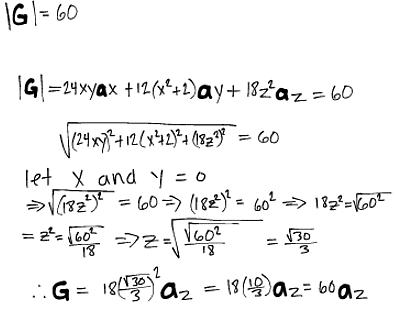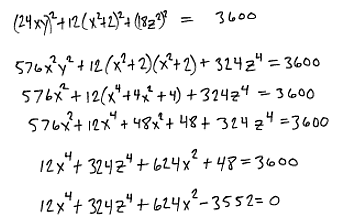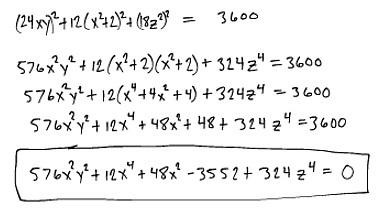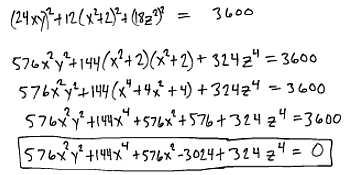# Find equation of surface with a given magnitude

Rombus

## Homework Statement

G=(24xy,12(x^2+2),18z^2)
|G|=60
Find the equation of the surface on which |G| = 60
Also two points were mentioned P(1,2,-1) and Q(-2,1,3) not sure if they are relevant to this problem.

## The Attempt at a Solution

Is this correct?Last edited:

Mentor

## Homework Statement

G=(24xy,12(x^2+2),18z^2)
|G|=60
Find the equation of the surface on which |G| = 60
Also two points were mentioned P(1,2,-1) and Q(-2,1,3) not sure if they are relevant to this problem.

## The Attempt at a Solution

Is this correct?Your second equation in the attached image doesn't make much sense to me. The third equation makes more sense, but has an error. G apparently is a vector-valued function, so |G| is the square root of the sum of the squares of the components of G.

IOW, ## |G| = \sqrt{ (24xy)^2 + 12^2(x^2 + 2)^2 + (18z^2)^2}##

I would expand the three terms in the radical and see if they could be combined and simplified. Then set the simplified expression equal to 60, and that will give you the equation you need.

As far as points P and Q are concerned, how were they mentioned? It could be that you are supposed to say whether they are on the surface.

BTW, this seems pretty advanced for precalculus, so I am moving it to the calculus & beyond section.

Homework Helper
Dearly Missed
Your second equation in the attached image doesn't make much sense to me. The third equation makes more sense, but has an error. G apparently is a vector-valued function, so |G| is the square root of the sum of the squares of the components of G.

IOW, ## |G| = \sqrt{ (24xy)^2 + 12^2(x^2 + 2)^2 + (18z^2)^2}##

I would expand the three terms in the radical and see if they could be combined and simplified. Then set the simplified expression equal to 60, and that will give you the equation you need.

It is easier to set ##|G|^2 = 60^2 = 3600,## since that gets rid of square roots. You would need to worry about making sure you have ##|G| = 60## rather than ##|G| = -60,## but that is likely easier to deal with than the ugly square roots.

RGV

Avatrin
You have not found the equation for a surface; You have found a point at which |G| is 60 (ie (0,0,60))).
|G| is equal to 60 at two points: (0,0,60) and (0,60,0).
Somehow you have to find the equation of a surface using this information. I think we need more details.

Homework Helper
Dearly Missed
You have not found the equation for a surface; You have found a point at which |G| is 60 (ie (0,0,60))).
|G| is equal to 60 at two points: (0,0,60) and (0,60,0).
Somehow you have to find the equation of a surface using this information. I think we need more details.

I think the information is sufficient: |G| = 60 gives a relationship between x, y and z, so defines one or more surfaces in E^3 (the different surfaces corresponding to different roots). Giving two points on the surface helps to pick out which root is correct.

RGV

Rombus
Thanks for the replies.
Is this the equation I'm looking for?

154910[/ATTACH]"]edit: looks like I lost my y^2 along the way.#### Attachments

Last edited:
Mentor
You have the same mistake you made in your first post. The second component of your vector-valued function is 12(x2 + 2). You are forgetting to square the 12.

Rombus
You have the same mistake you made in your first post. The second component of your vector-valued function is 12(x2 + 2). You are forgetting to square the 12.

So after fixing that mistake is this the equation I'm looking for? Thanks again.Mentor
That looks more like it, but the first line is still wrong. The middle term in the left side should be 122(x2 + 2)2.

Also, rather than post a photo of your work, it's better to type it into the input pane. If you click Go Advanced, you get an expanded menu of capabilities (including exponents and subscripts), plus a menu of common symbols that appears to the right.

Rombus
Ok, thank you, and thanks for the tip about posting.